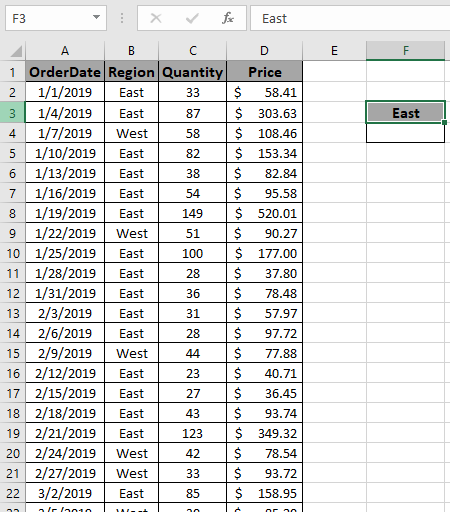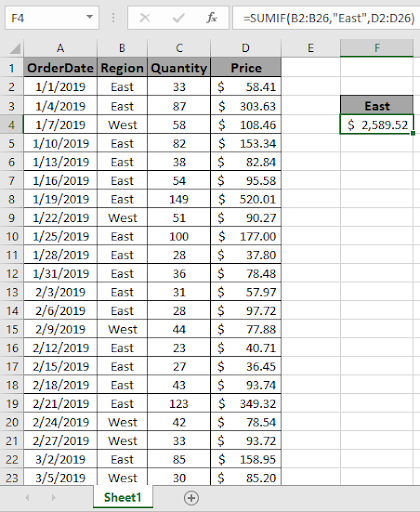# Sum if cell is equal to value

In this article, we will learn about how to get the SUM value if cell value is equal to a specific value in Excel.

In simple words, when working with long data sheets, sometimes we need to extract the sum of values where cell is equal to the value like getting the sum of monthly expense where just a specific day’s amount is required.

Let’s get the Generic Formula:

=SUMIF (range, “value”, sum_range)

range : condition on the array
value : value to match
sum_range : range where sum is needed.

Let’s understand this function using it in an example.

Here we have Order from different regions with their respective quantity and Price.We need to get the total Price for region East.

Use the formula:

=SUMIF(B2:B26,"East",D2:D26)

Explanation:
B2:B26 : range where formula matches values.
"East" : the formula now accepts all East.
A2:A26 : sum_range where amount adds up.

The formula drops down to

=SUMIF(
{"East";"East";"West";"East";"East";"East";"East";"West";"East";"East";
"East";"East";"East";"West";"East";"East";"East";"East";"West";"West";"East";"West";"East";
"East";"West"},
"East",
{58.41;303.63;108.46;153.34;82.84;95.58;520.01;90.27;177;37.8;78.48;57.97;97.72;
77.88;40.71;36.45;93.74;349.32;78.54;93.72;158.95;85.2;107.97;139.6;160.82})
This formula adds up all the values corresponding to all the values “East”.As you can see this formula returns the sum ( \$ 2589.52 ) if cell is equal to a value (East).
Hope you understood how to get the SUM if cell is equal to value in Excel. Explore more articles on Excel SUMIF function here. Please feel free to state your query or feedback for the above article.
We need to get the total Price for region East.

Terms and Conditions of use

The applications/code on this site are distributed as is and without warranties or liability. In no event shall the owner of the copyrights, or the authors of the applications/code be liable for any loss of profit, any problems or any damage resulting from the use or evaluation of the applications/code.